• Shuffle
Toggle On
Toggle Off
• Alphabetize
Toggle On
Toggle Off
• Front First
Toggle On
Toggle Off
• Both Sides
Toggle On
Toggle Off
Toggle On
Toggle Off
Front

## Card Range To Study

throughPlay buttonPlay buttonProgress

1/99

Click to flip

Use LEFT and RIGHT arrow keys to navigate between flashcards;

Use UP and DOWN arrow keys to flip the card;

H to show hint;

 A push or pull on a system. Can either be scalar or vector. Force The SI unit of force. Newton The force that holds protons and neutrons together in nuclei. Strong nuclear force The attractive or repulsive force produced by static and moving charges in atoms. Electromagnetic force A force exerted between elementary particles of matter within the nucleons weak nuclear force The force exerted by all matter on other matter. gravitational force The force that acts between systems when one system touches another. contact force The mathematical description of a field force exerted in the space surrounding an object that is the source of or is susceptible to the field force. field A non contact force exerted on a susceptible object by similar objects. field force A force that in combination with other forces acting on the same system produces a zero net force on the system. balanced force A force exerted on a system by something in its surroundings. external force The single unbalanced force acting on a system that is the sum of all forces acting on the system. net force A force that in combination with other forces acting on the same system results in a nonzero net force and produces a change in motion of the system. unbalanced force The tendency of all matter to resist change in motion. inertia Written by Newton and define the science of dynamics and apply to all areas of mechanics. Laws of motion A law stating that objects at rest remain at rest and objects in motion continue in a straight line at a constant velocity unless acted upon by a net external force. law of inertia (Newton's first law) The condition in which all forces acting on a system are balanced as indicated by the system's lack of acceleration. mechanical equilibrium Law stating that the acceleration of a system is directly proportional to the net force acting on the system and is inversely proportional to the system's mass. Law of accelerated motion (Newton's second law) Law stating that for every force exerted on a system by its surroundings, the system exerts an equal but opposite force. action-reaction principle (Newton's third law) A contact force that opposes the movement of objects past each other. friction The force exerted by a supporting surface on a object resting on it. normal force Law stating that the force of gravity between two objects is directly proportional to the products of their masses and inversely proportional to the square of the distance between their centers of mass. Law of universal gravitation The acceleration of an object due to gravity. At the earth's surface its average magnitude is about 9.81 m/s/s, regardless of the object's mass. gravitational acceleration The acceleration of an object accelerated by the force of gravity alone with no other forces acting on it. free fall A constant acceleration, such as that of an object in free fall. uniform acceleration A form of friction exerted by a fluid on an object moving in reaction to the fluid. drag The maximum constant speed of an object falling in the atmosphere. terminal velocity A property of a moving object directly proportional to its mass and speed momentum The momentum of a system moving in a straight line; a quantity equal to the product of the system's mass and velocity. linear momentum The momentum of a rotating system, proportional to the mass of the system, the speed at which it rotates, and the direction of the mass in relation to the axis of rotation. angular momentum The change of a system's momentum, directly proportional to the force exerted and the time interval over which the force is applied. impulse A fundamental conservation law of mechanics stating that in a system of colliding objects, the sum of their momentums before the collision is equal to the sum of their momentums afterward if no external force acts upon the objects. conservation of momentum The natural tendency of all moving things is to ____ and ______. slow down stop When an outside force acts on an object it will continue in its original state of motion. (T or F) F What is a force? A push or pull on a system. Is a force scalar or vector? either A force must act upon a system to change the object's ______. velocity The force remaining after all other forces have been taken into account is called a(n)____________. Unbalanced force Forces transmitted when two objects are touching are called _______________. Contact forces List four examples of forces identified in Q6 Compression Tension Torsion Shear Forces that act without physical contact are called ______________. field forces List three examples of the forces identified in Q8 magnetic gravitational electrostatic The first law of motion is sometimes called _________________. The Law of Inertia The property of matter that causes objects to resist change in the state of motion is called ____________. inertia Every object has inertia (T or F) T More mass means less inertia (T or F) F Mass does not affect inertia (T or F) F The unit of force is a(n) _______ Newton What is the unit of force? a Newton How do you measure force (ex: in for length) kg-m/s/s F=ma is a mathematical statement of which law? Newton's second law Which law explains why a fully loaded dump truck is harder to accelerate than the same empty one? The second law one unit of force gives _____ kilogram of mass an acceleration of ____ meter per second squared. (put #s in the blanks) one one (directly proportional) How can the third law of motion be described? For every action there is an equal and opposite reaction. and/or If one object gains momentum, then the other object must lose an equal amount of momentum and in the opposite direction. Force is _______proportional to acceleration. directly Forces always come (by themselves) or (in pairs) in pairs.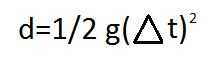What is this the formula for? the distance of a falling object What is terminal velocity? The limit of the velocity at which an object can fall through a gas or liquid. Why doesn't a falling object keep accelerating? other forces interfere. What are the four fundamental forces in order of strongest to weakest? strong nuclear forces electrostatic forces weak nuclear forces gravity Of the four fundamental forces which force is the weakest? gravity The nearer the mass the weaker its gravity (T or F) F The greater the mass the stronger the gravity (T or F) T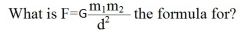gravitational force What is the unit for mass? kg What is the unit for weight? N Define weight. The measure of the force of gravity acting on a mass. What is w=km the formula for? weight What is a Newton? The force required to give 1kg of mass an acceleration of 1m/s/s Does your weight vary depending on where you are on earth? yes What instrument is used to measure mass? balance What instrument is used to measure weight? spring scale What is the unit for the force of attraction between two objects? N What is p=mv the formula for? momentum What are the units for momentum? kg-m/s If both the mass and velocity of an object are relatively small, the momentum will be relatively ______. small If the momentum is large, then the mass and velocity will be relatively ________. large Which has a higher momentum: a car with a 5000kg mass moving with a velocity of 5 m/s a bike with a 50kg mass moving with a velocity of 500m/s. they're equal Which has a higher momentum? An apple with a 3 kg mass rolling with a velocity of 15m/s A beach ball with a 10 kg mass rolling with a velocity of 4 m/s The apple Does momentum transfer from one object to another? What is this process known as? yes The conservation of momentum What is an example for the conservation of momentum? the firing of a rifle The conservation of momentum states that momentum can be ________ from one object to another without a change in _____ _________ of the system. transferred total momentum Is it possible for a 70kg man to have a greater momentum that an 250kg man? Why? yes The 70kg man has to have a velocity high enough to overcome his mass. When two objects of unequal mass collide, the lighter object is accelerated by the collision to a much (faster/slower) speed than the heavier object faster Identify the two factors that work together to effect a change in momentum. force applied time applied What is impulse? A changing in momentum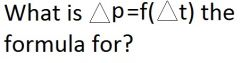Impulse What is E=mb the formula for? linear momentum Which of the four fundamental forces is the strongest? Strong Nuclear force What is p=mv the formula for? momentum What is V=d/t the formula for? speedacceleration What is f=ma the formula for? Newton's 2nd lawdistance of a falling object what is w=mg the formula for? weight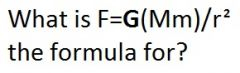universal gravity btwn two objectsimpulse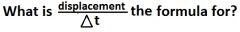velocity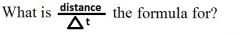speed